•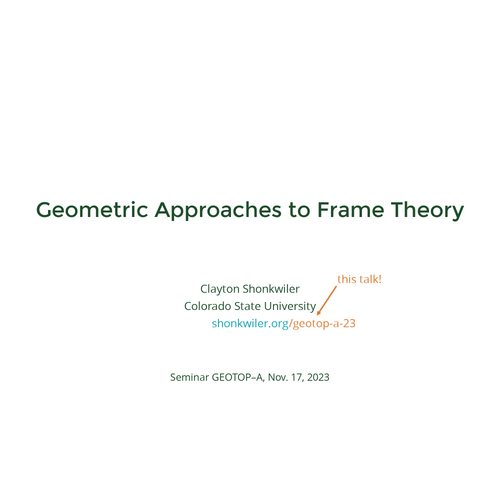## Geometric Approaches to Frame Theory

•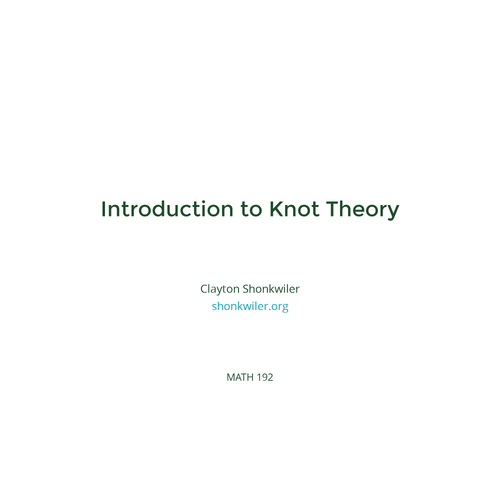## Introduction to Knot Theory

•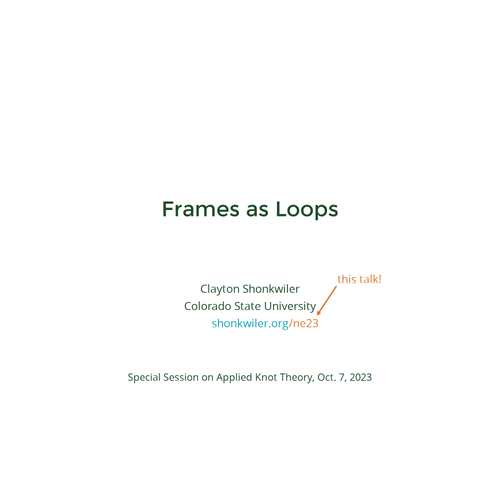## Frames as Loops

•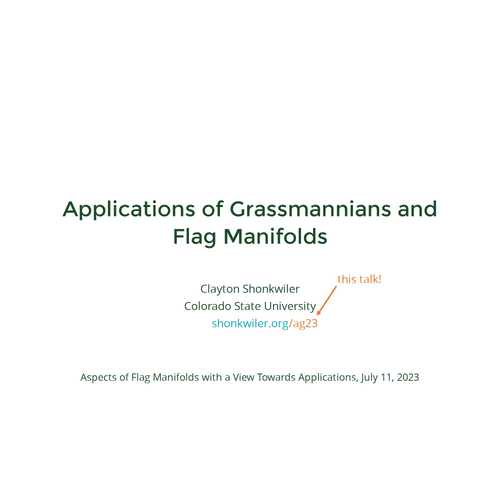## Applications of Grassmannians and Flag Manifolds

•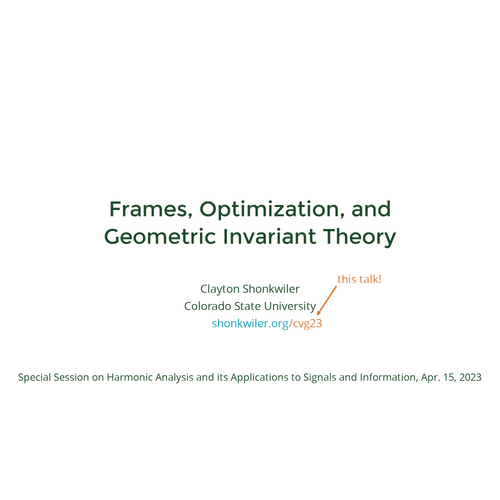## Frames, Optimization, and Geometric Invariant Theory

•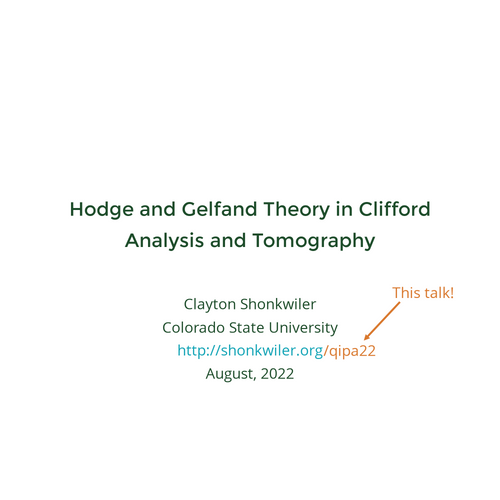## Hodge and Gelfand Theory in Clifford Analysis and Tomography

•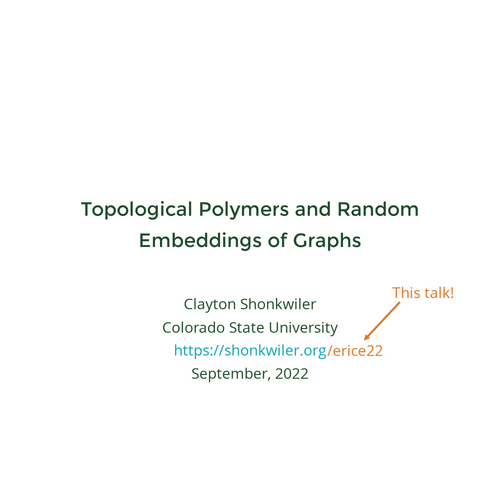## Topological Polymers and Random Embeddings of Graphs

•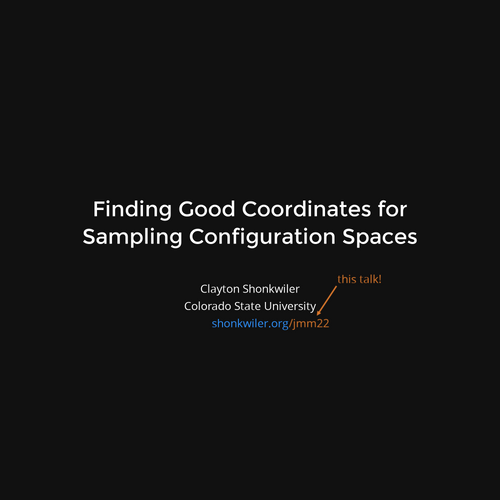## Finding Good Coordinates for Sampling Configuration Spaces

•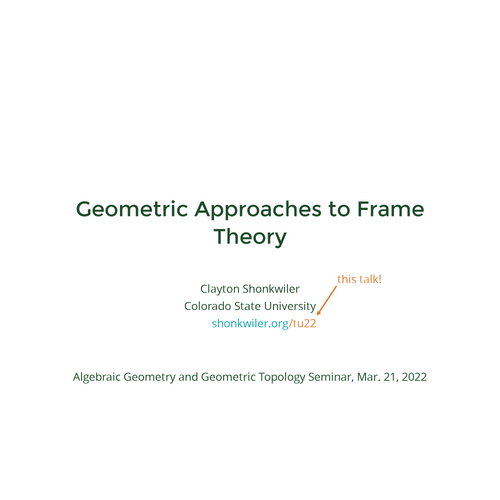## Geometric Approaches to Frame Theory

•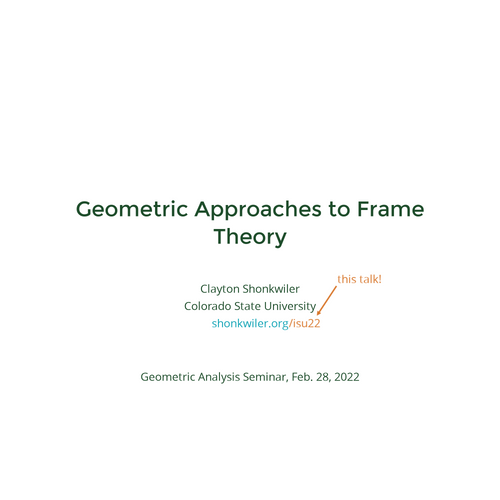## Geometric Approaches to Frame Theory

•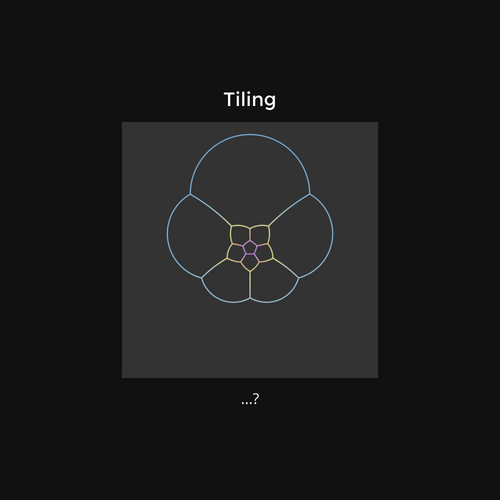## Animations

•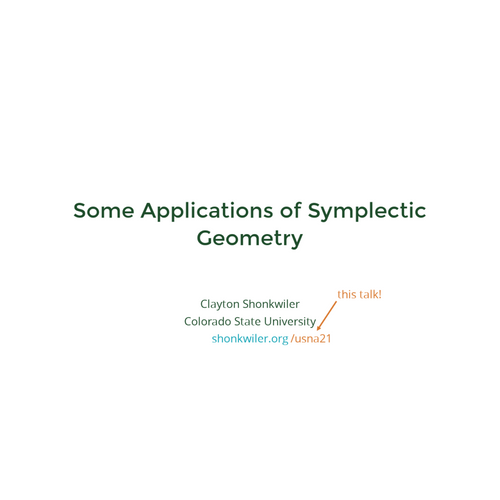## Some Applications of Symplectic Geometry

•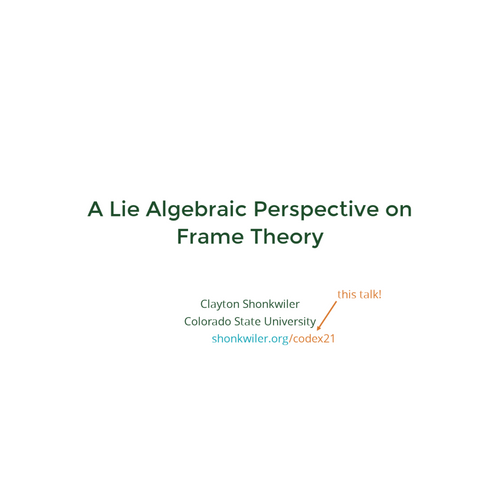## A Lie Algebraic Perspective on Frame Theory

•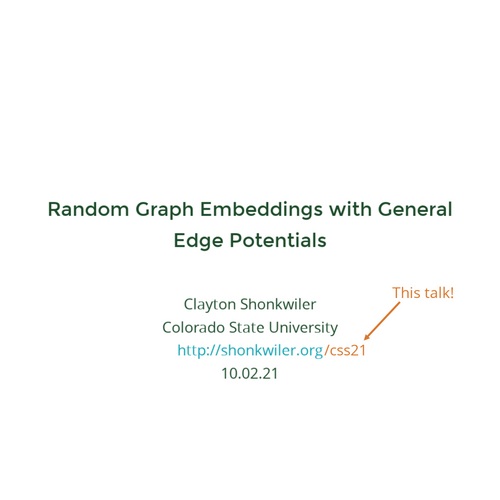## Random Graph Embeddings with General Edge Potentials

•## What is a Random Knot? And Why Do We Care?

•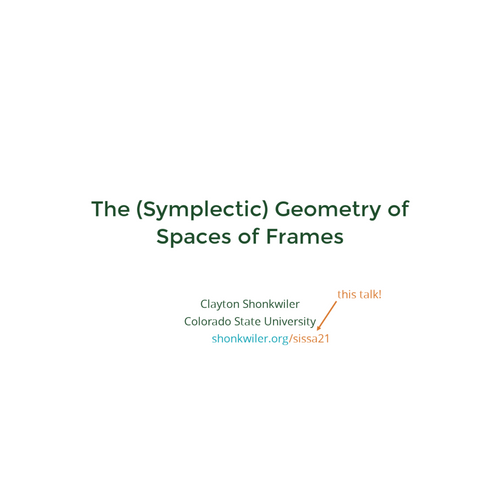## The (Symplectic) Geometry of Spaces of Frames

•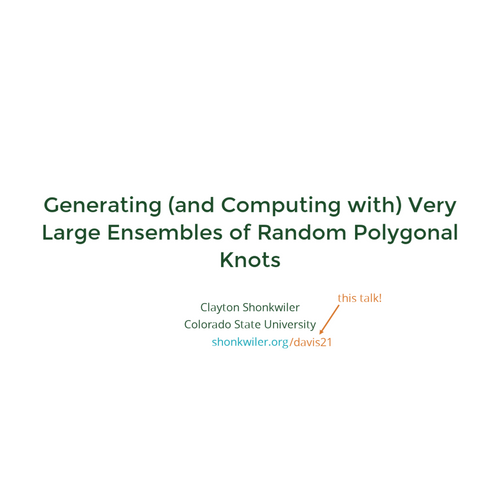## Generating (and Computing with) Very Large Ensembles of Random Polygonal Knots

•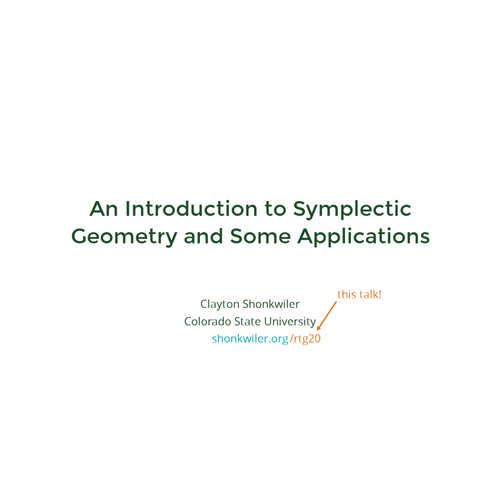## An Introduction to Symplectic Geometry and Some Applications

•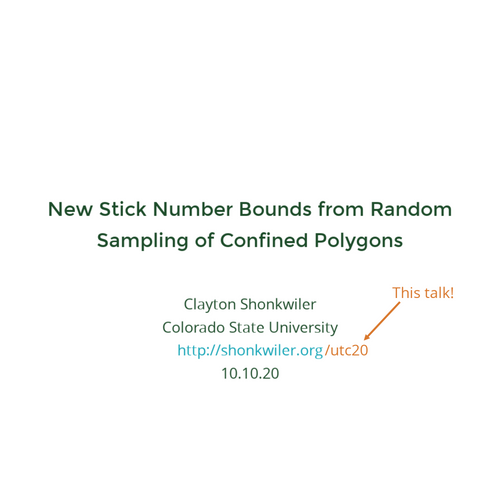## New Stick Number Bounds from Random Sampling of Confined Polygons

•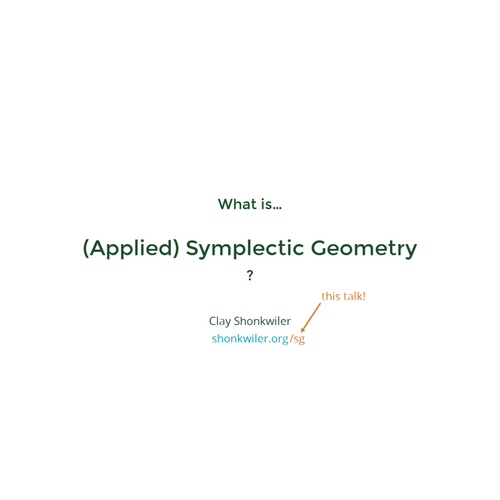## What is (Applied) Symplectic Geometry?

•## New Stick Number Bounds from Random Sampling of Confined Polygons

•## Hamiltonian Group Actions on Frame Spaces

•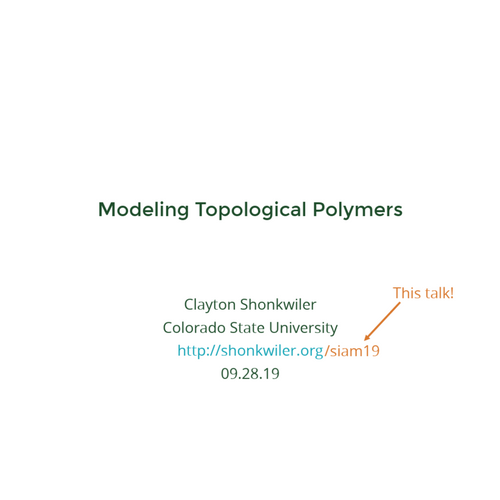## Modeling Topological Polymers

•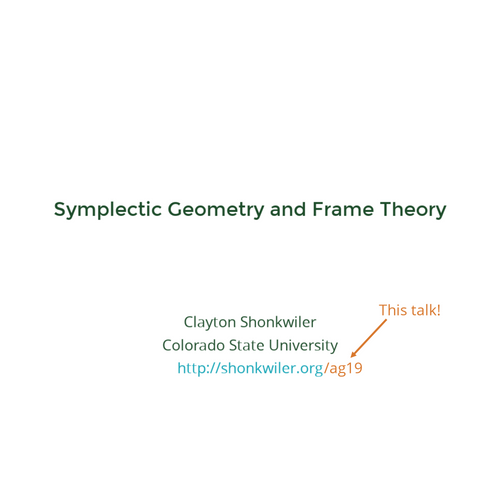## Symplectic Geometry and Frame Theory

•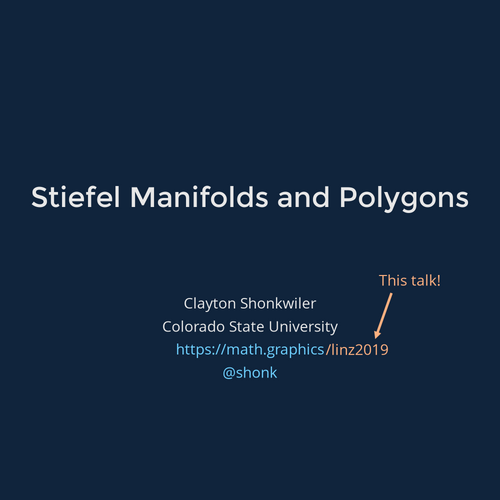## Stiefel Manifolds and Polygons

•## Visualizing Higher Dimensions

•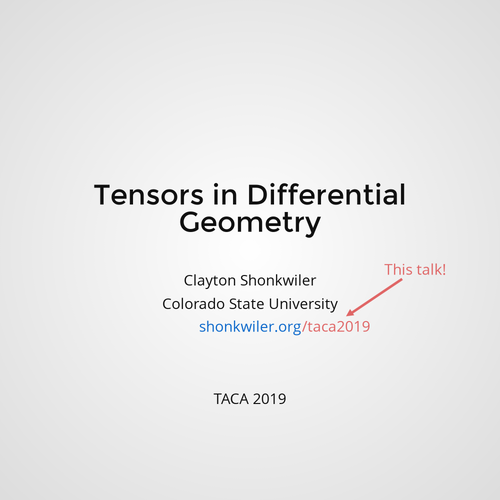## Tensors in Differential Geometry

•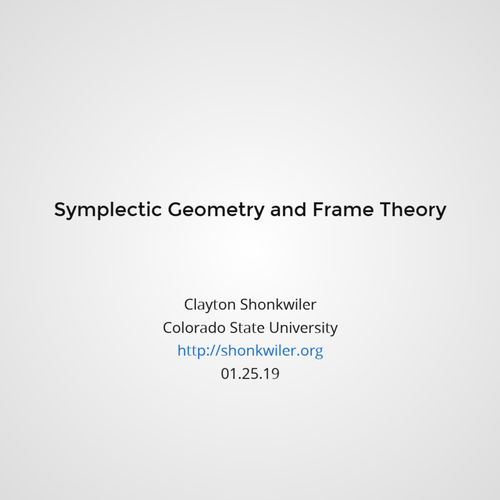## Symplectic Geometry and Frame Theory

•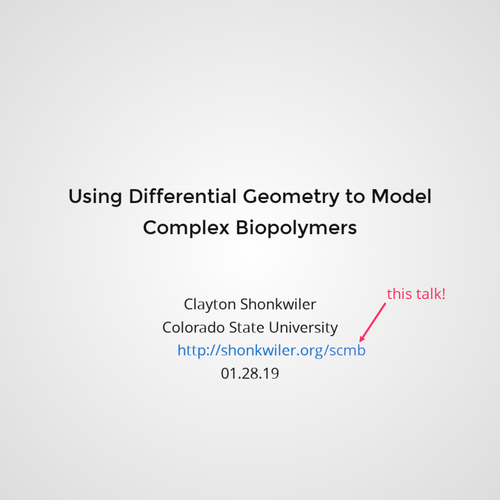## Using Differential Geometry to Model Complex Biopolymers

•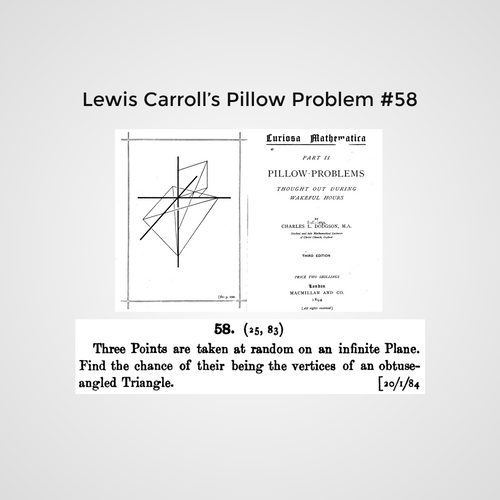## What’s the Probability a Random Triangle is Obtuse?

For MATH 161

•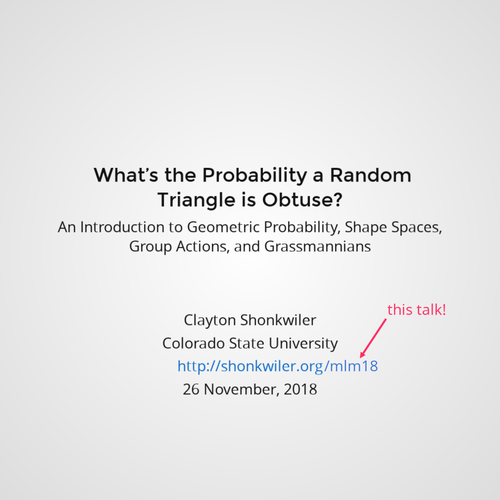## What’s the Probability a Random Triangle is Obtuse?

An Introduction to Geometric Probability, Shape Spaces, Group Actions, and Grassmannians

•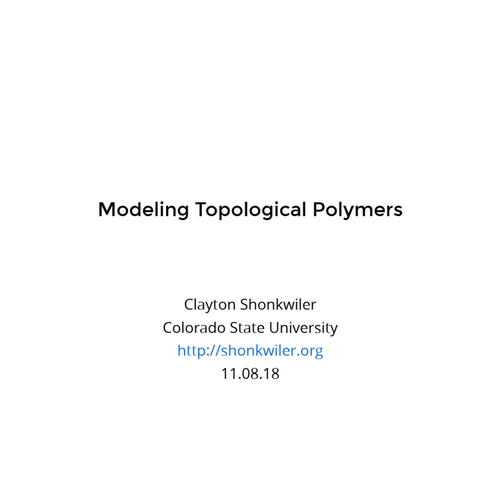## Modeling Topological Polymers

•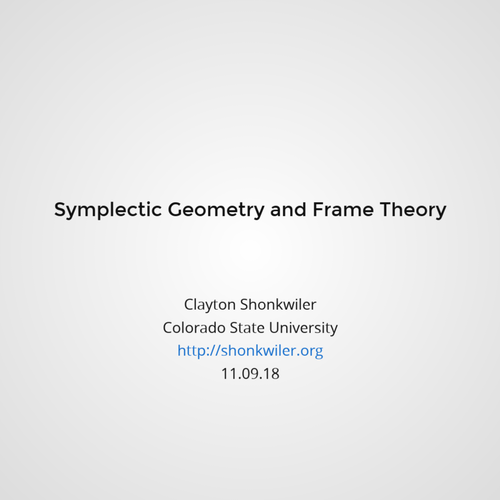## Symplectic Geometry and Frame Theory

•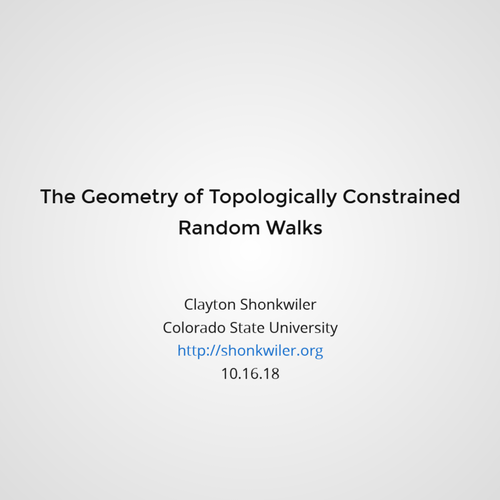## The Geometry of Topologically Constrained Random Walks

•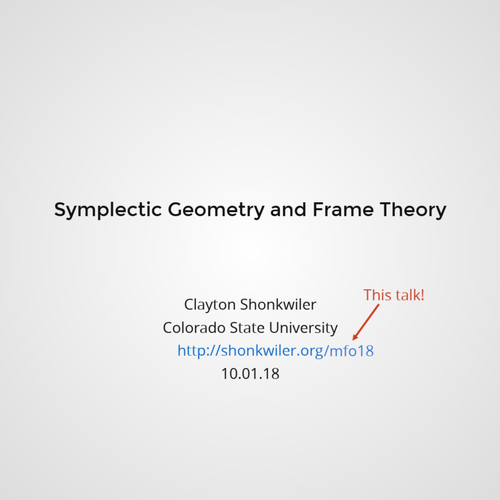## Symplectic Geometry and Frame Theory

•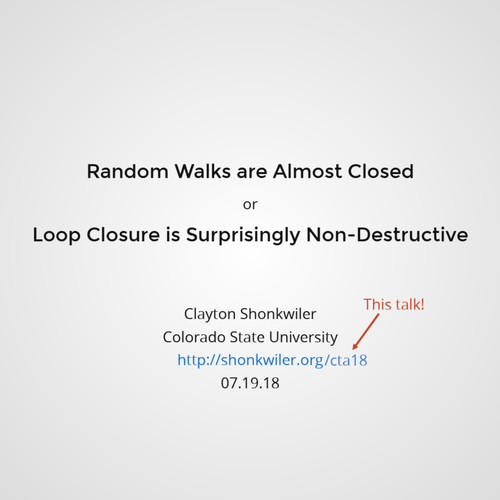## Random Walks are Almost Closed

Loop closure is surprisingly non-destructive

•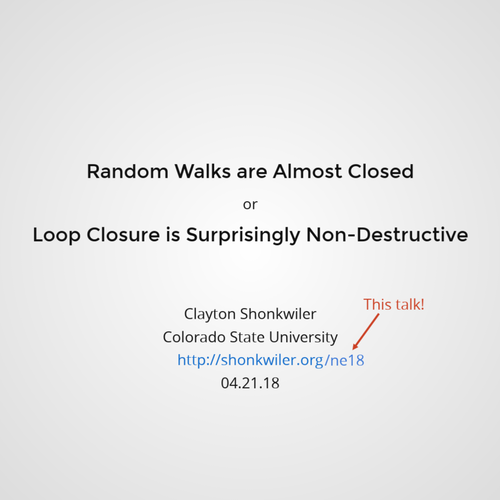## Random Walks are Almost Closed

Loop closure is surprisingly non-destructive

•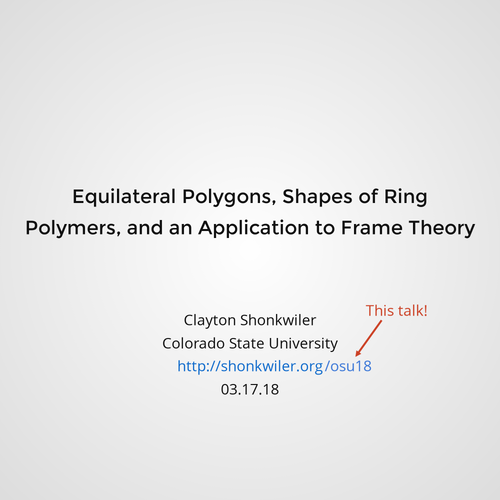## Equilateral Polygons, Shapes of Ring Polymers, and an Application to Frame Theory

Random flights in 3-space forming a closed loop, or random polygons, are a standard simplified model of so-called ring polymers like bacterial DNA. Equilateral random polygons, where all steps are the same length, are particularly interesting (and challenging) in this context. In this talk I will describe an (almost) toric Kähler structure on the moduli space of equilateral polygons and show how to exploit this structure to get a fast algorithm to directly sample the space. Using work of Hausmann–Knutson, the Kähler structure on the space of equilateral polygons can be realized as the Kähler reduction of the standard Kähler structure on the Grassmannian of 2-planes in complex n-space. This means that equilateral polygons in 3-space can be lifted to Finite Unit-Norm Tight Frames (FUNTFs) in complex 2-space. I will describe how to modify the polygon sampler to produce a FUNTF sampler and show that optimal packings in the 2-sphere lift to FUNTFs with low coherence.

•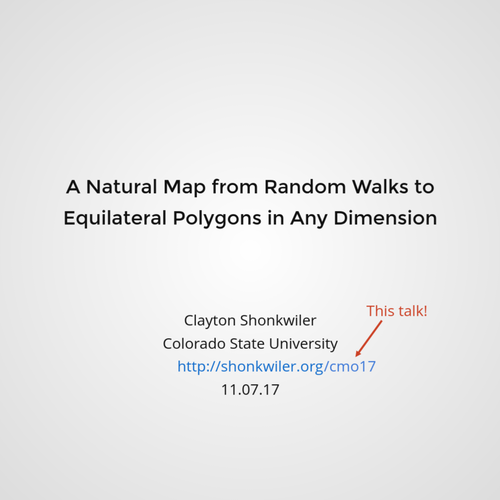## From random walks to closed polygons

A natural map from random walks to equilateral polygons in any dimension

•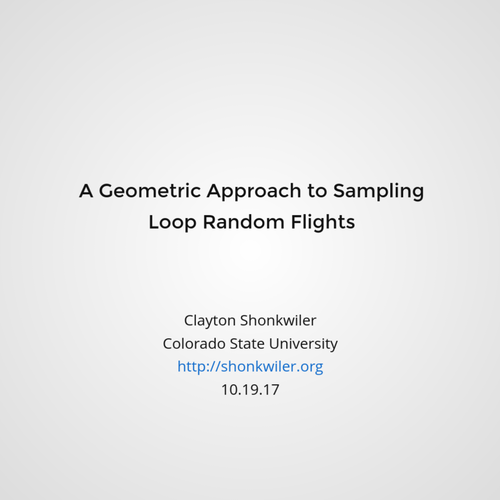## A Geometric Approach to Sampling Loop Random Flights

In statistical physics, the basic (and highly idealized) model of a ring polymer like bacterial DNA is a closed random flight in 3-space with equal-length steps, often called an equilateral random polygon. While random flights without the closure condition are easy to simulate and analyze, the fact that the steps in a random polygon are not independent has made it challenging to develop practical yet provably correct sampling and numerical integration techniques for polygons. In this talk I will describe a geometric approach to the study of random polygons which overcomes these challenges. The symplectic geometry of the space of polygon conformations can be exploited to produce both Markov chain and direct sampling algorithms; in fact, this approach can be generalized to produce a sampling theory for arbitrary toric symplectic manifolds. This is joint work with Jason Cantarella, Bertrand Duplantier, and Erica Uehara.

•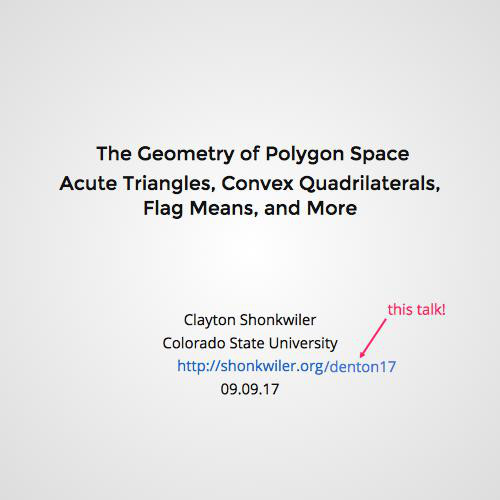## The Geometry of Polygon Space

Acute triangles, convex quadrilaterals, flag means, and more

•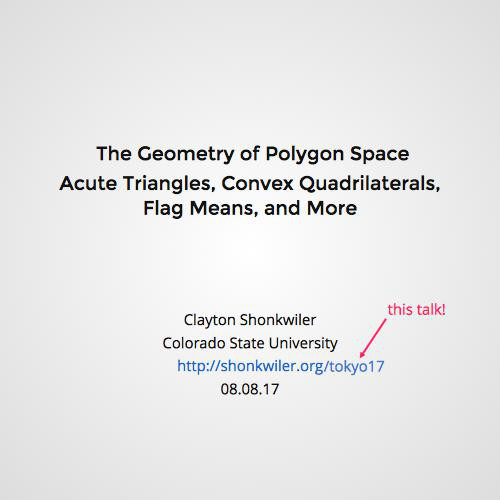## The Geometry of Polygon Space

Acute triangles, convex quadrilaterals, flag means, and more

•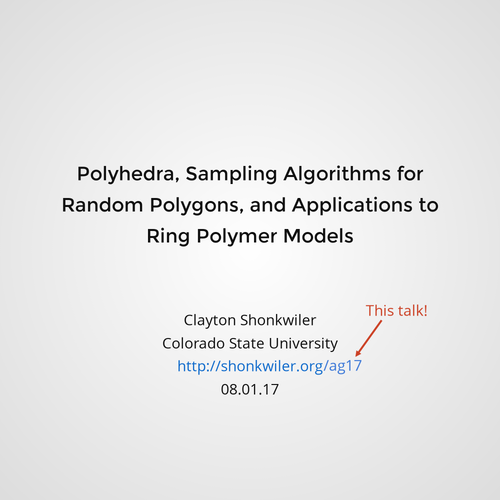## Polytopes and Polygons

Polyhedra, sampling algorithms for random polygons, and applications to ring polymer models

•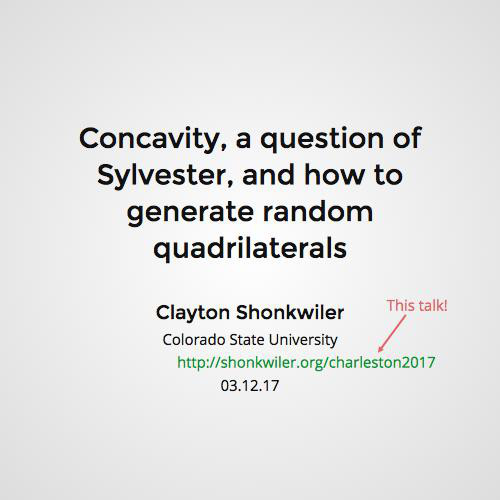Concavity, a question of Sylvester, and how to generate random quadrilaterals

•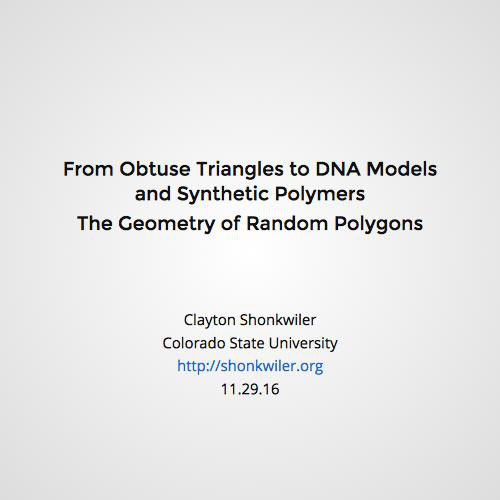## The Geometry of Random Polygons

From obtuse triangles to DNA models and synthetic polymers

•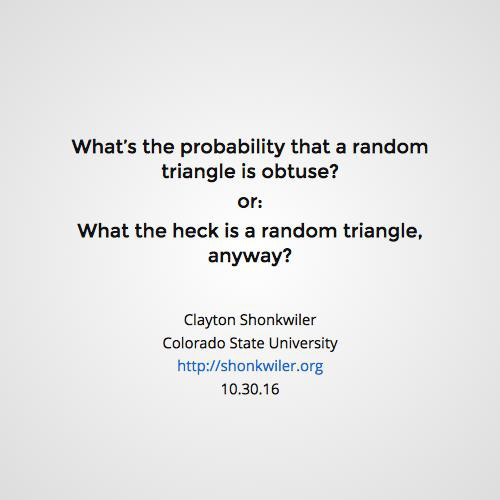## Random Triangles

What's the probability that a random triangle is obtuse? Or, what the heck is a random triangle, anyway?

•## Random Triangles

What's the probability that a random triangle is obtuse? Or, what the heck is a random triangle, anyway?

•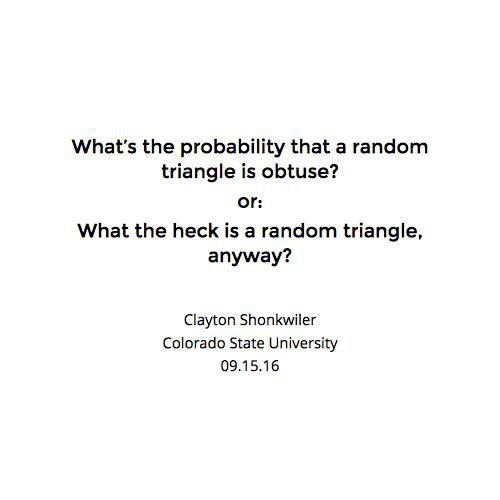## Random Triangles

What's the probability that a random triangle is obtuse? Or, what the heck is a random triangle, anyway?

•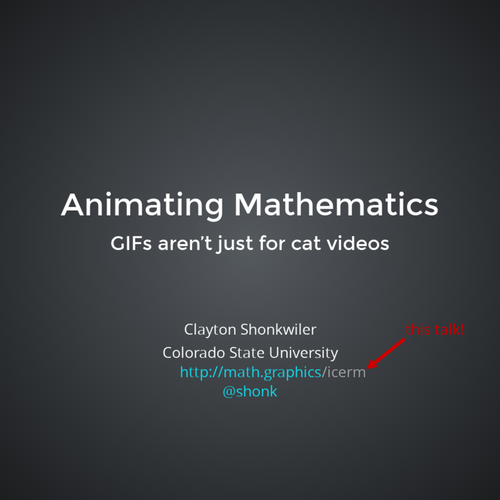## Animating Mathematics

The animated GIF, which provided flashing lights and rotating "Under Construction" tackiness to the early web, has had an unlikely second life on social media. Aside from serving as a medium for sharing video while ducking the copyright cops, GIFs are embraced by a generation of abstract and geometric artists on Tumblr and elsewhere who have discovered beauty in short, looping animations. My first GIFs were created to sanity-check algorithms and I have since used them in both the classroom and the seminar room, but I also now create mathematical animations that are purely artistic. In this talk I will share some of my GIFs and the (trivial and non-trivial) mathematics behind them. My work is produced in Mathematica with help from a couple command-line tools, so I will also try to impart some hard-earned lessons on how to use this combination to produce GIFs that (probably) won't make you want to claw out your eyeballs.